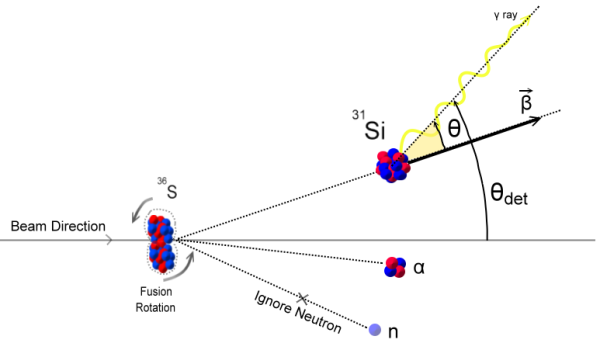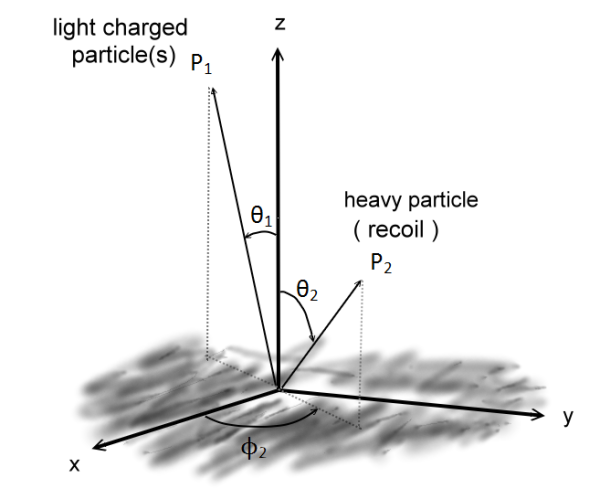# Kinematic reconstruction:

Here is our situation, and let me explain this situation in the lab frame. Suppose we create a projectile (beam) to hit our static target, and it temporarily forms a compound, and then it separates to other types of elements, including a heavier recoil and several lighter particles.

Usually, we detect the energies and velocities of these lighter particles, and that is what a particle detector is designed for. And Our goal is to get the energies and velocities of the heavier recoil. We use the linear momentum conservation law to solve this problem, and if you wish, we can do it in either the lab frame or the center mass frame.Figure: a demonstration of our problem setup. We wish to use the information of the lighter particles and the conservation of linear momentum law to acquire the energies and velocities of the heavier recoil.

You can superpose all the linear momentum vectors of the lighter particles. Then the system after the reaction will be just two vectors; one is for heavier recoil, and the other one is the superposition of the lighter particles. It forms a binary system.Figure: the coordinate setup.

In the above equation, P is for the momentum. Before the reaction, we only have projectile moving, and it is the initial momentum Pbeam. The subscript 1 for the lighter particles, and the subscript 2 for the heavier recoil. After some manipulation, we can get: